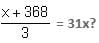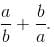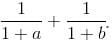CAT  >  Practice Questions Level 1: Quadratic Equations & Linear Equations - 1

# Practice Questions Level 1: Quadratic Equations & Linear Equations - 1 - Notes | Study Level-wise Practice Questions for CAT Preparation - CAT

 1 Crore+ students have signed up on EduRev. Have you?

This EduRev document offers 10 Multiple Choice Questions (MCQs) from the topic Quadratic Equations & Linear Equations (Level - 1). These questions are of Level - 1 difficulty and will assist you in the preparation of CAT & other MBA exams. You can practice/attempt these CAT Multiple Choice Questions (MCQs) and check the explanations for a better understanding of the topic.

Question for Practice Questions Level 1: Quadratic Equations & Linear Equations - 1
Try yourself:Total integer pair(s) (x, y) satisfying the equation x + y = xy is/are

Question for Practice Questions Level 1: Quadratic Equations & Linear Equations - 1
Try yourself:If A = 2 and B + P + F = 24, what are the values of Q and S? Consider whole numbers only.
A + B = Z, Z + P = T, T + A = F, F + S = Q, Q - T = 7

Question for Practice Questions Level 1: Quadratic Equations & Linear Equations - 1
Try yourself:Which of the following is the correct value of x for the equationQuestion for Practice Questions Level 1: Quadratic Equations & Linear Equations - 1
Try yourself:Let x and y be real numbers, such that (x2 - y2)(x2 - 2xy + y2) = 3 and x - y = 1. What is the value of xy?

Question for Practice Questions Level 1: Quadratic Equations & Linear Equations - 1
Try yourself:If p, q and r are the roots of the cubic equation x3 - 3x2 + 5x + k = 2 and pqr = 1, then find the value of k.

Question for Practice Questions Level 1: Quadratic Equations & Linear Equations - 1
Try yourself:Find the value of x3 + y3 + z3 - 3xyz when x + y + z = 9 and xy + yz + zx = 11.

Question for Practice Questions Level 1: Quadratic Equations & Linear Equations - 1
Try yourself:If (a2 + b2)3 = (a3 + b3)2, then find the value ofQuestion for Practice Questions Level 1: Quadratic Equations & Linear Equations - 1
Try yourself:1/2 (a + b + c){(a - b)2 + (b - c)2 + (c - a)2} is equal to

Question for Practice Questions Level 1: Quadratic Equations & Linear Equations - 1
Try yourself:What is the value of y if  1/x + 2/y = 3/z ?

Question for Practice Questions Level 1: Quadratic Equations & Linear Equations - 1
Try yourself:If x = ay and y = bx, where the values of x and y cannot be zero, find the value ofThe document Practice Questions Level 1: Quadratic Equations & Linear Equations - 1 - Notes | Study Level-wise Practice Questions for CAT Preparation - CAT is a part of the CAT Course Level-wise Practice Questions for CAT Preparation.
All you need of CAT at this link: CAT

277 docs

## Level-wise Practice Questions for CAT Preparation

277 docs

### How to Prepare for CAT

Read our guide to prepare for CAT which is created by Toppers & the best Teachers

Track your progress, build streaks, highlight & save important lessons and more!

,

,

,

,

,

,

,

,

,

,

,

,

,

,

,

,

,

,

,

,

,

;